The repository is available at https://github.com/mjenrungrot/hmc-cs152.

# CS152 - Animated Style Learning by Teerapat (Mek) Jenrungrot

This project is a final project for CS 152 - Neural Network in Spring 2017 taught at Harvey Mudd College.

# Introduction

Image Style Learning

Style transfer problem has become very popular in the field of computer vision and image processing. As introduced by Image style transfer using convolutional neural networks, the authors have shown that using image representations derived from convolutional neural networks can explicitly represent semantic information and is able to separate image content from image style. The authors demonstrated their works by introducing a neural algorithm for constructing an image that has similar content to a reference content image and similar style to a reference style image. This idea of representing an image with deep neural networks, as demonstrated in Understanding deep image representations by inverting them, has been shown that several layers of deep convolutional neural networks can retain photographically accurate information about the image, with different degrees of geometric and photometric invariance.

Further using an idea of representing an image with deep convolutional neural networks, we are interested in a problem that, given a set of images known to be constructed according to the neural algorithm—presented by Image style transfer using convolutional neural networks— what is a style image illustrating the style of images in the dataset. Then, we can arbitrarily combine any input image with that style image to create a new image that replicates the style of images in the dataset. As shown in Figure 1, we are interested in investigating a neural algorithm that can learn to construct a style image given a dataset of images with similar style.

The scope of this final project is to generate a style image that can be regarded as a representative of Japanese animated-style image. The expected generated images should be applicable to the neural algorithm from Image style transfer using convolutional neural networks such that given any input image we can make it have a style like Japanese animation artwork, such as the image shown in Figure 1.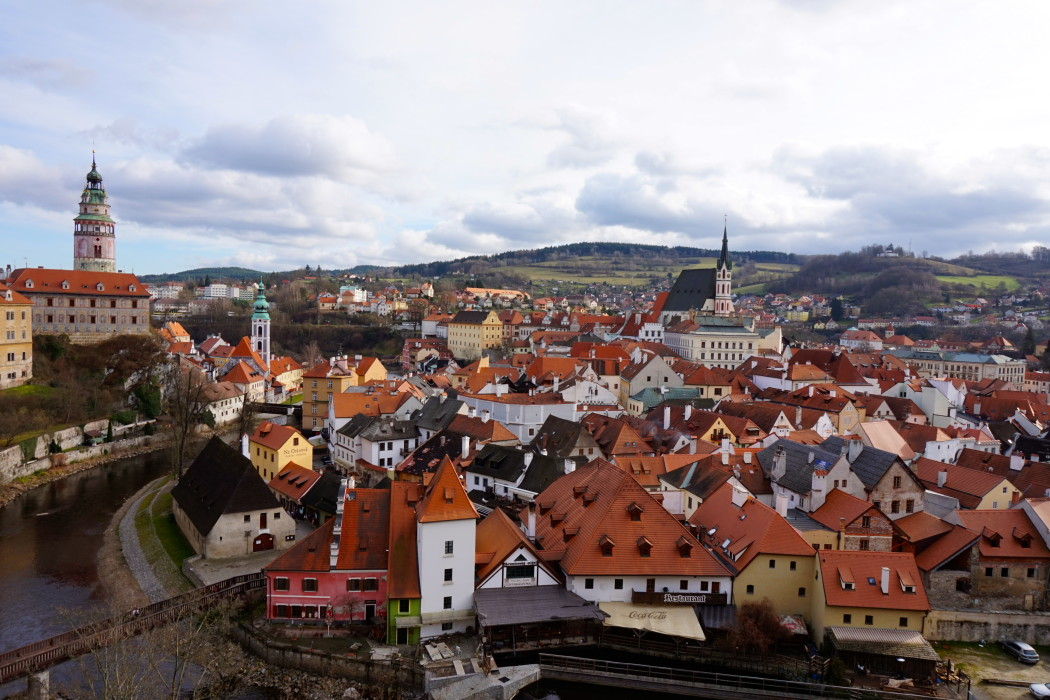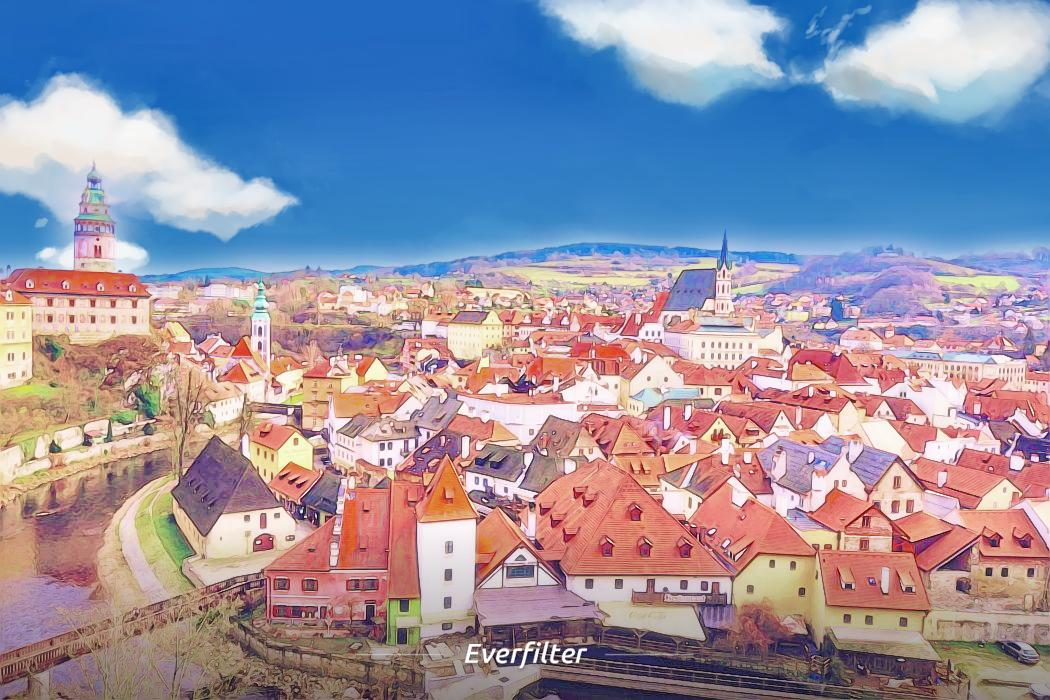Figure 1: A comparison between the reference content image and an image generated by the algorithm. The right image is generated by an online image conversion tools EverFilter

# Previous Works

Image style transfer using convolutional neural networks

In transferring style problem, the goal is to synthesize a new image that contains both semantic content information from the reference content image and artistic style information from the reference style image. As demonstrated in Figure 2, an output image is generated based on a reference content image depicting the Tübinger, Germany, and a reference style image entitled The Shipwreck of the Minotaur by J.M.W. Turner, 1805. The generated image is able to illustrate the content information similar to the reference content image of the Tübinge while also render the style information similar to the reference style image The Shipwreck of the Minotaur.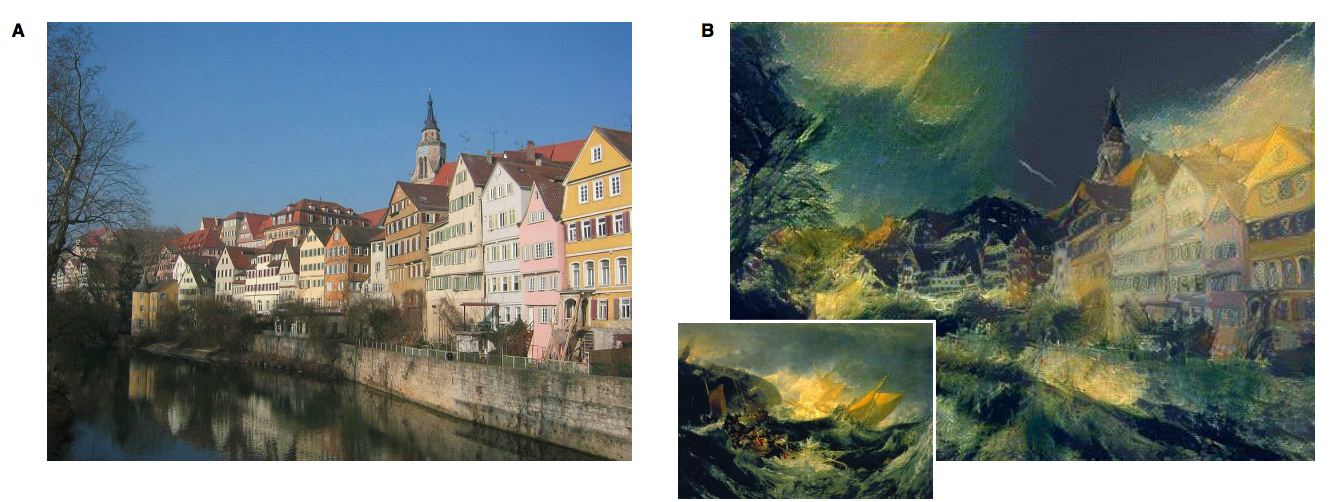Figure 2: an example of results produced by a neural algorithm described in Image style transfer using convolutional neural networks. Left: a content image depicting the Neckarfront in Tübinger, Germany; Right: a transformed image generated by the algorithm; Bottom: the painting named The Shipwreck of the Minotaur by J.M.W. Turner, 1805. This example is taken from Image style transfer using convolutional neural networks.

The definition of style and content of an image is ambiguous. Because we do not have precise definitions of what portions/qualities of an image contributes to "style" or "content" of an image. These two terms are also vaguely defined, depending on individuals. Before moving forward, we define a few definitions regarding the similarity between images' style and content.

Suppose $\vec{p}$ represent a content image vector, $\vec{s}$ represent a style image vector, and $\vec{x}$ represent any input image vector. Consider a convolutional neural network that will be used to represent features of an image. In this paper, the authors use VGG network with 19 layers, in short VGG-19 network. Suppose layers used to represent a style image is $\mathcal{S}$ and layers used to represent a content image is $\mathcal{C}$.

Definition 1: Let $P^l$ and $X^l$ be features' representations of a content image $\vec{p}$ and an input image $\vec{x}$ on layer $l$ of a trained network. Two images have similar content information if their high-level features obtained by the trained classifier have small Euclidean distance. In other words, two images have similar style if the following loss function regarding content representation of two images is small:

$\mathcal{L}_\text{content}(\vec{p},\vec{x}) = \sum\limits_{l\in\mathcal{C}} \left[ \frac{1}{U_l} \sum\limits_{i,j} \left(P_{ij}^{l} - X_{ij}^{l}\right)^2 \right]$

where $X_{ij}^l$ and $P_{ij}^{l}$ are the feature representations on layer $l$ of $i^\text{th}$ filter at position $j$ of an input image and a content image, respectively, as represented by the trained classifier. The term $U_l$ is the total number of units on layer $l$, used for the purpose of weighting contribution of each layer.

Definition 2: Let $S^l$ and $X^l$ be representations of a style image $\vec{s}$ and an input image $\vec{x}$ on layer $l$ of a trained network. Two images have similar style if the difference between their features' Gram matrices has a small Frobenius norm. The Gram matrix, $G_\vec{x}^l$, of any image $\vec{x}$ on layer $l$ is defined as follows:

$G_\vec{x}^l = \left[ G_{\vec{x},ij}^l \right]$

where each element of the Gram matrix is given by inner products between feature representations on the trained classifier on layer $l$ for any two different filters:

$G_{\vec{x},ij}^{l} = \sum\limits_{k}X^{l}_{ik}X_{jk}^l$.

In the same way, two images have similar style if the following loss function regarding style representation of two images is small.

$\mathcal{L}_\text{style}\left(\vec{s},\vec{x}\right) = \sum\limits_{l \in \mathcal{S}} \left[ \frac{1}{U_l} \sum\limits_{i,j}\left( G_{\vec{s},ij}^l - G_{\vec{x},ij}^l \right)^2 \right]$

where $G_{\vec{s},ij}^l$ and $G_{\vec{x},ij}^l$ are elements at $i^\text{th}$ row and $j^\text{th}$ column of Gram matrices for a style image $\vec{s}$ and an input image $\vec{x}$ based on feature representations in the trained classifier on layer $l$.

Algorithm

In Image style transfer using convolutional neural networks, a deep convolutional neural network (VGG-19) is used as a trained classifier for obtaining feature representations of any input images, content images, and style images. VGG-19 network is a classifier trained for solving image recognition task on over 10 millions images. The set of layers used for representing content information is $\mathcal{C} = \{\texttt{conv4_2}\}$. The set of layers used for representing style information is $\mathcal{S} = \{\texttt{conv2_1}, \texttt{conv3_1}, \texttt{conv4_1}, \texttt{conv5_1}\}$.

More details about the structure of VGG network is available at Very Deep Convolutional Networks for Large-Scale Image Recognition. The main advantages of using the VGG network, compared to other convolutional neural network, are 1) able to run on a large scale image recognition settings, 2) generalizable with different datasets, and 3) representable with deep networks. The generalizability over different kind of datsets and its deep network structure make it possible for each layer to retain photographically accurate information, with different degree of variation. Specifically, the deeper layer of the network is responsible for global structure of an image, while the shallower layer of the network is responsible for fine structure of an image, such as strokes or edges. Note that the VGG network is the winner of the ImageNet Large-Scale Visual Recognition Challenge (ILSVRC-2012). The dataset ImageNet contains over 10 millions of images with various kind of objects.

Given a reference style image $\vec{s}$ and a reference content image $\vec{p}$, the algorithm starts by putting these two images into the trained classifier VGG-19 network in order to obtain feature representations on each layer of the VGG-19 network. For style features, the Gram matrix on each layer $l \in \{\texttt{conv2_1}, \texttt{conv3_1}, \texttt{conv4_1}, \texttt{conv5_1}\}$ is calculated and stored. For content features, the feature representation on layer $l \in \{\texttt{conv4_2}\}$ is stored. Once we have all feature representations of reference images, we then try to generate an image $\vec{x}$ that minimizes the following loss function:

$\mathcal{L}_\text{total}(\vec{p},\vec{s},\vec{x}) = \alpha \mathcal{L}_\text{content}(\vec{p},\vec{x}) + \beta \mathcal{L}_\text{style}(\vec{s},\vec{x})$

where $\alpha$ and $\beta$ are weighting factors for content and style reconstruction, respectively. The learning algorithm will then optimize $\vec{x}$ such that it minimizes $\mathcal{L}_\text{total}(\vec{p},\vec{s},\vec{x})$ using gradient descent algorithm, such as L-BFGS. In the process of optimizing loss function, we start with a randomized white noise. This randomized white noise is put into a trained classifier VGG-19 network to obtain feature representations in each layer of the network. The Gram matrix is also calculated accordingly in the same manner. Next, the loss function is calculated. Since the loss function is differentiable, we then follow the gradient descent algorithm to optimize an input image $\vec{x}$ that optimizes the total loss function $\mathcal{L}_\text{total}(\vec{p},\vec{s},\vec{x})$.

# Animated Style Learning

Background

When human perceives any image, one can usually recognize the type of that image, for instance, if it is a drawing, painting, photograph, etc. Oftentimes, one with enough background knowledge can further indicate the specific kind of image. For example, one can specifically indicate that an image is an artwork from a specific animated film drawn by a specific artist. The idea that people can recognize the specific type of images conceptualizes an idea of recognizing style of an image.

For this final project, we are interested in investigating what do we mean by recognizing style of an image. Specifically, we consider Japanese animated-style artworks artificially generated by an application. We want to explore if there exists any universal representation of any specific kind of artworks, such as artworks from Japanese animation domain.

In this project, we consider using an application named EverFilter to generate Japanese animated-style artworks. EverFilter is an application that can transform any input image into works of animated art. The producers of EverFilter claim that they apply similar algorithm to the one introduced by Image style transfer using convolutional neural networks. We are interested in reversing the process of what EverFilter does. That is, given images generated by EverFilter, we want to find what corresponding style image is. The scope of this project is illustrated in Figure 3.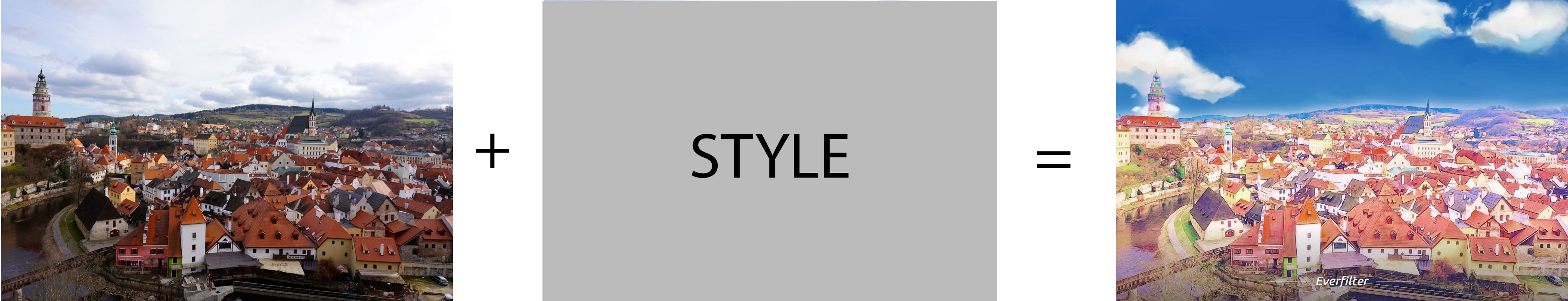Figure 3: An illustration of the scope for this project

Approach

In this section, we describe our approach on constructing a style image. Recall the definition of style similarity mentioned above that two images have similar style if and only if the difference between features' Gram matrices has a small Frobenius norm. Here, we re-modeled the objective function so that it excluded the cost function due to content loss. The justification is that content information should take no effect in obtaining style information. Using a similar method of image reconstruction, we start with a randomized white noise and optimize the cost function using backpropagation to generate a style image.

We believe that the generated style image should be generalizable across different type of input images. So, we decide to construct a style image based on many reference EverFilter's transformed images. The modified objective function of our approach then becomes:

$\mathcal{L}(\texttt{dataset},\vec{x}) = \sum\limits_{i=1; \vec{s} \in \texttt{dataset}}^{|\texttt{dataset}|}\alpha_{i}\mathcal{L}_\text{style}(\vec{s},\vec{x})$

where $\vec{s}$ and $\vec{x}$ is a style image vector and an input image vector, $\alpha_i$ is a weighting parameter of each reference style image, and $\texttt{dataset}$ is a dataset of EverFilter's transformed images. Images in the dataset approximately gathered manually by the author. Here is the link to all images in the dataset [Link].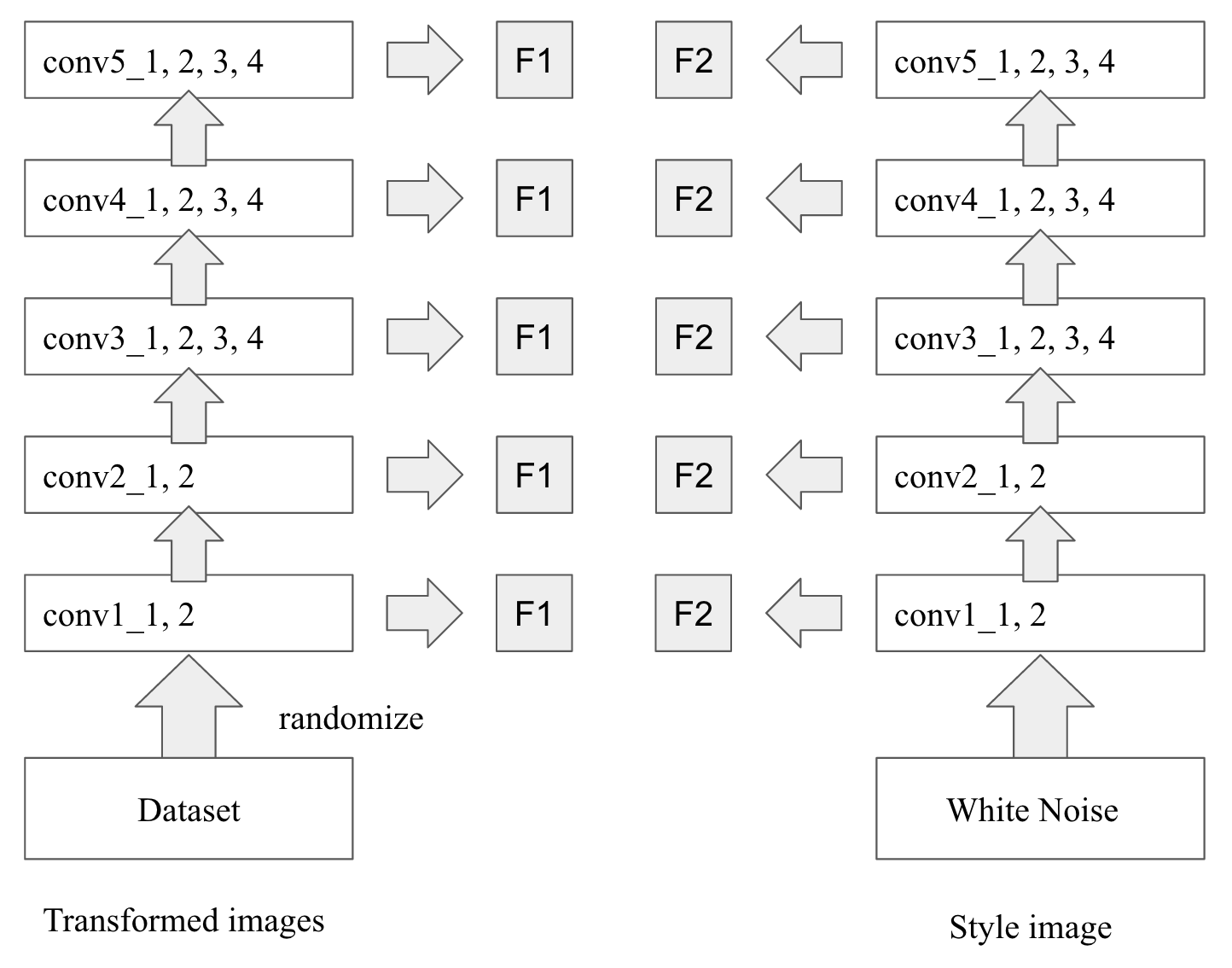Figure 4: The structure of our proposed algorithm. At every iteration, we randomize images from the dataset, then input to the VGG-19 pre-trained network. We then calculate the Gram's matrix, F1, from the result of pre-trained network. To create a style image, we input to same VGG-19 pre-trained network in order to compute the Gram's matrix, F2, in the similar manner. We then update the style image using the back-propagation method to minimize the Frobenius norm between F1 and F2.

Figure 4 shows the workflow of our approach. We first obtain the pre-trained model of VGG-19 model. Next, we applied the pre-trained VGG-19 model on all images in the dataset to obtain feature representations on all layers. Note that this is slightly different from the style transfer algorithm we described in earlier section. According to Improving the neural algorithm of artistic style, using all layers to represent a style image rather than using only some layers works better. So, we decided to use all layers to represent style information. Next, we then start with a randomized white noise image as an input image. The input image is put into the pre-trained VGG-19 model. Feature representations on all layers of the trained classifier are extracted to compute corresponding Gram's matrices. Then, the objective function is calculated accordingly. The input image is then updated iteratively acccording to backpropagation algorithm to get an image that minimizes the loss function described earlier.

Since the concept of style is vaguely established, it is possible that an image can have multiple styles on different portion of an image. As one may notice in Figure 3, the background portion depicting a sky is, arguably, not having the same style as the forground portion depicting a town scene. Different portions of an image in the dataset seem to have different feature distributions. To address this consideration, we also experiment applying a masking technique so that we only consider a particular portion of an image to calculate related a Gram matrix. By applying the masking technique on one area of a reference style image, the style image should be able to generate a new image whose style is similar to its reference in the masking area.

# Results & Discussion

In this project, we ran all the testings and optimizations on the Pittsburgh Supercomputing Center, which is a high performance computing center. The specification of a GPU node I used is HPE Apollo 2000s, each with 2 NVIDIA P100 GPUs, 2 Intel Xeon E5-2683 v4 CPUs (16 cores per CPU), 128GB RAM, and 8TB on-node storage. The graphics card is a NVIDIA P100 GPU which has Memory Clock of 1.4Gbps and VRAM of 12GB.

Recall our proposed objective function.

$\mathcal{L}(\texttt{dataset},\vec{x}) = \sum\limits_{i=1; \vec{s} \in \texttt{dataset}}^{|\texttt{dataset}|}\alpha_{i}\mathcal{L}_\text{style}(\vec{s},\vec{x})$

We ran 3 experiments using our proposed method. The first experiment involves using only a single reference file for the dataset in the above equation. The second experiment involves using multiple reference files for the dataset. The third experiment involves using a single reference file for the dataset and a masking technique to generate a background portion and a foreground portion of an image separately. We present results and discussions of each experiment separately.

1st Attempt: Dataset of size 1, without masking

First, we tested our approach for generating a style image according to our proposed loss function for a dataset of size 1. Figure 5, Figure 6, and Figure 7 show results generated based on 3 diffrerent reference files. We have included the generated image at different epochs to illustrate what the algorithm learn to optimize.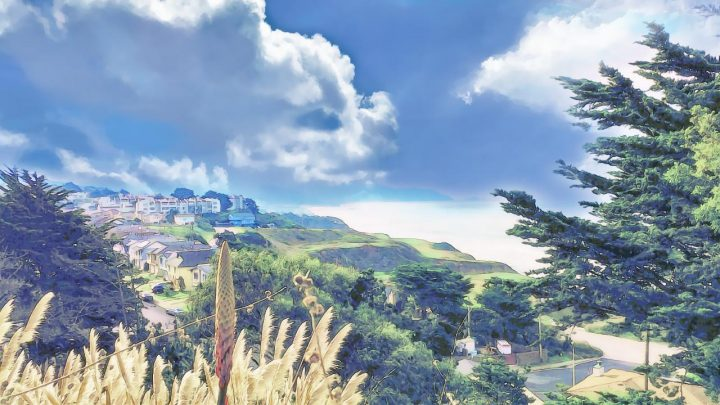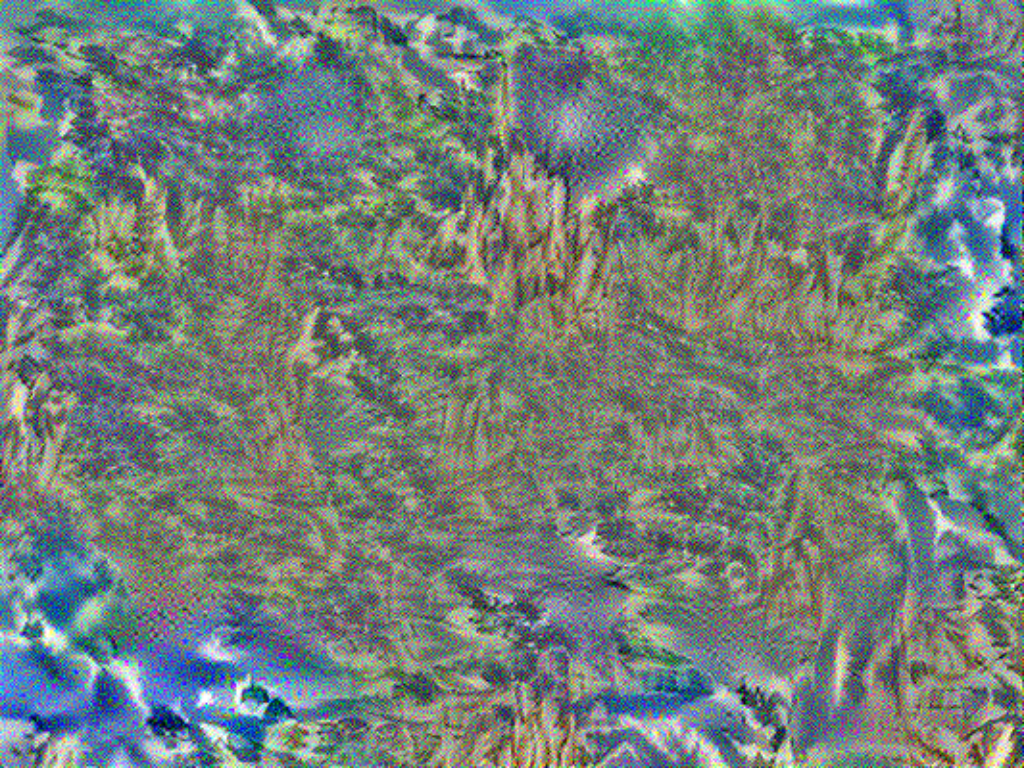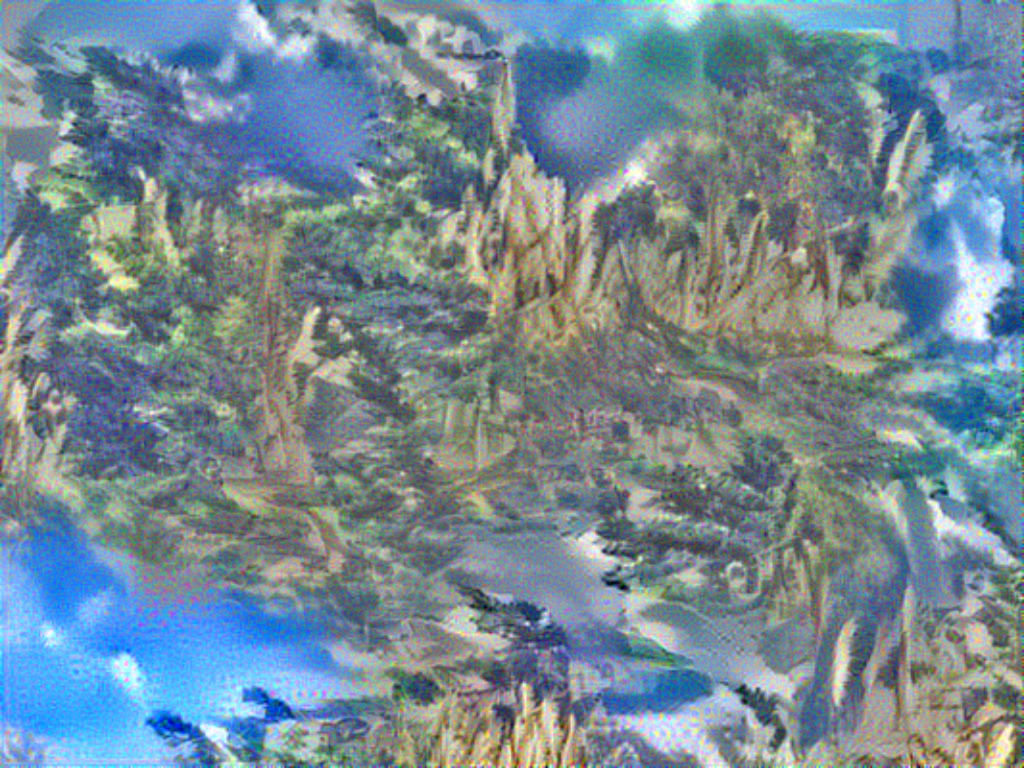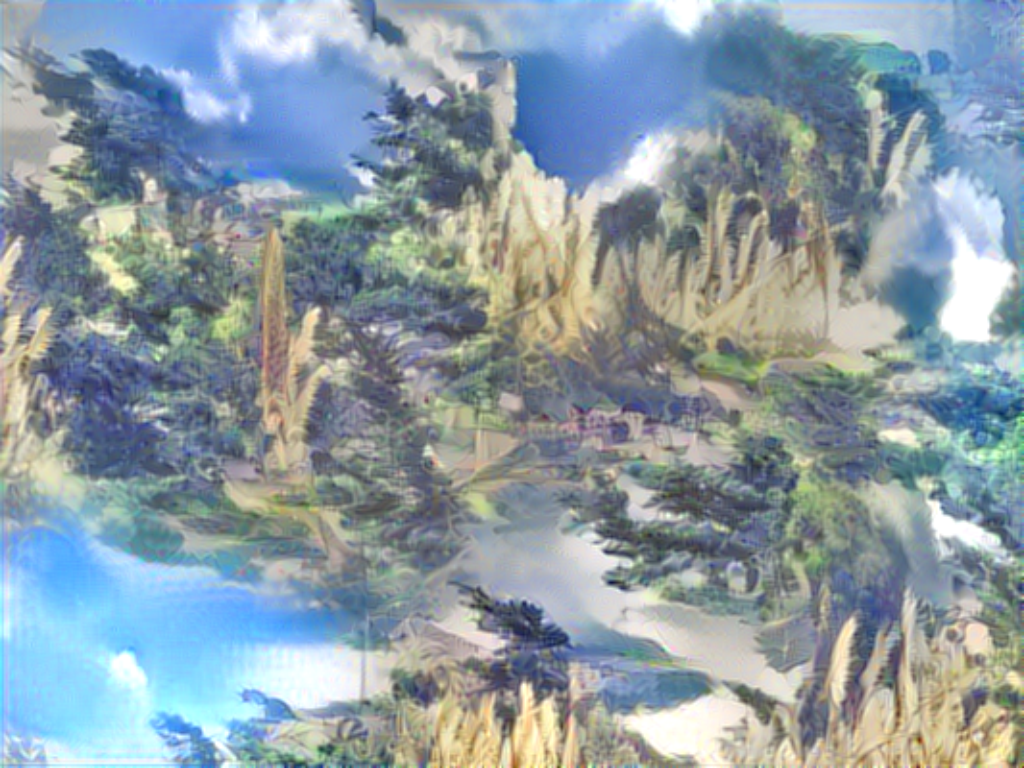Figure 5: An example of output from the algorithm for the dataset of size one. Top-left: the reference transformed image; Top-right: the generated image after 15 epochs; Bottom-left: the generated image after 150 epochs; Bottom-right: the generated image after 1500 epochs.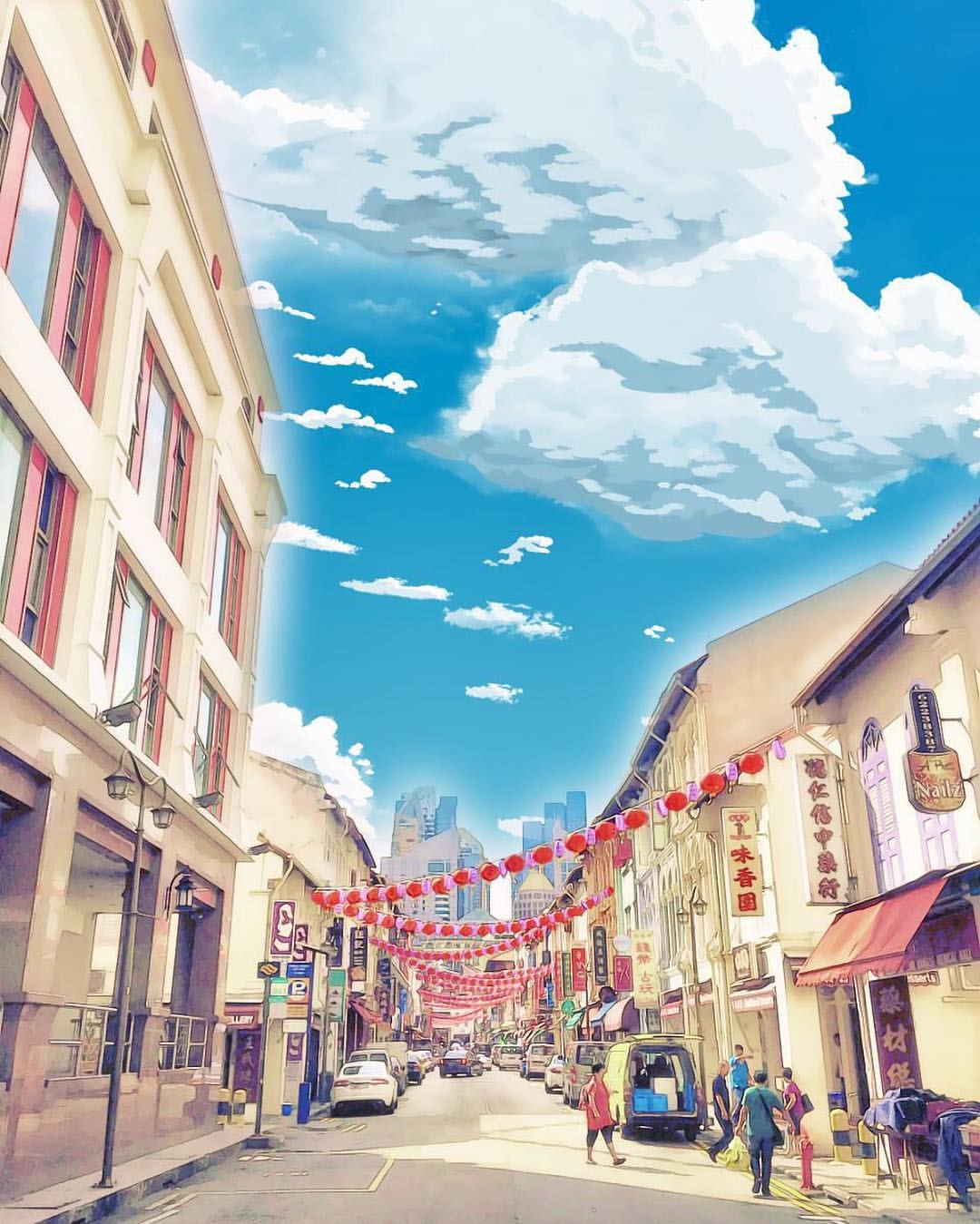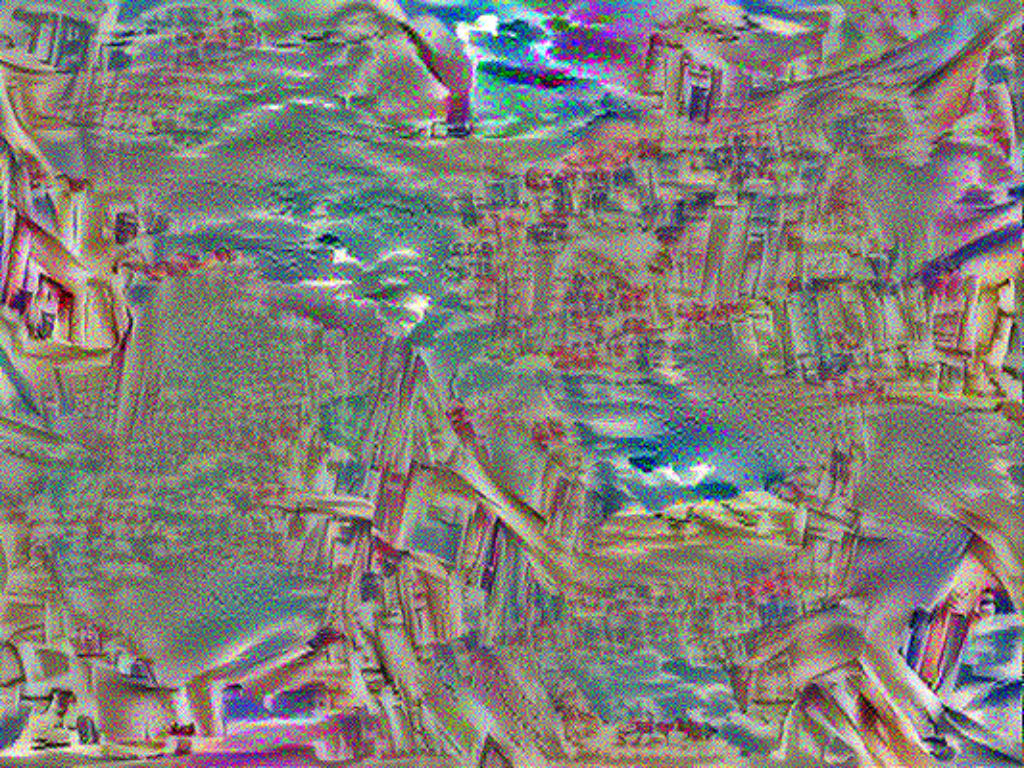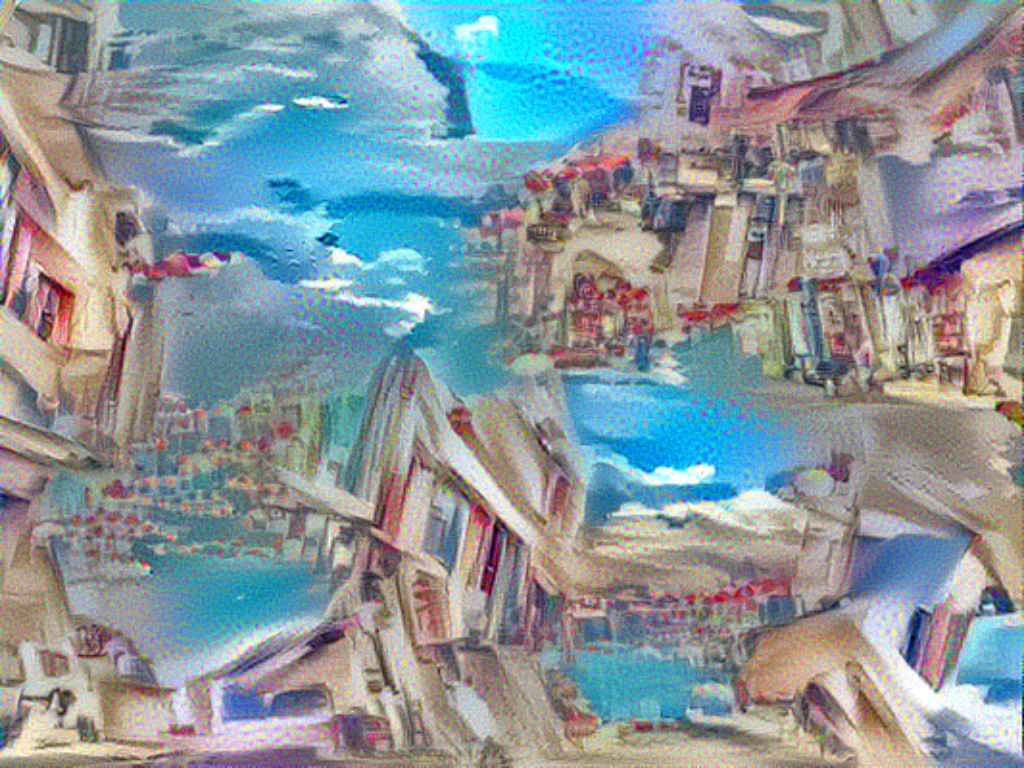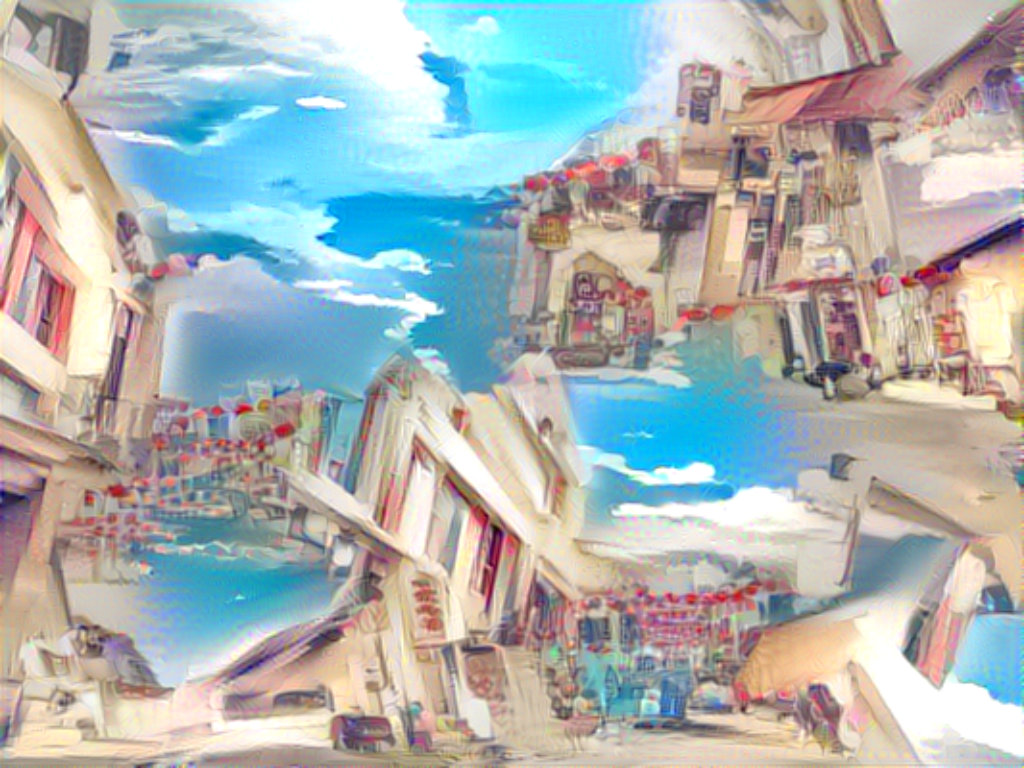Figure 6: Another example of output from the algorithm for the dataset of size one. Top-left: the reference transformed image; Top-right: the generated image after 15 epochs; Bottom-left: the generated image after 150 epochs; Bottom-right: the generated image after 1500 epochs.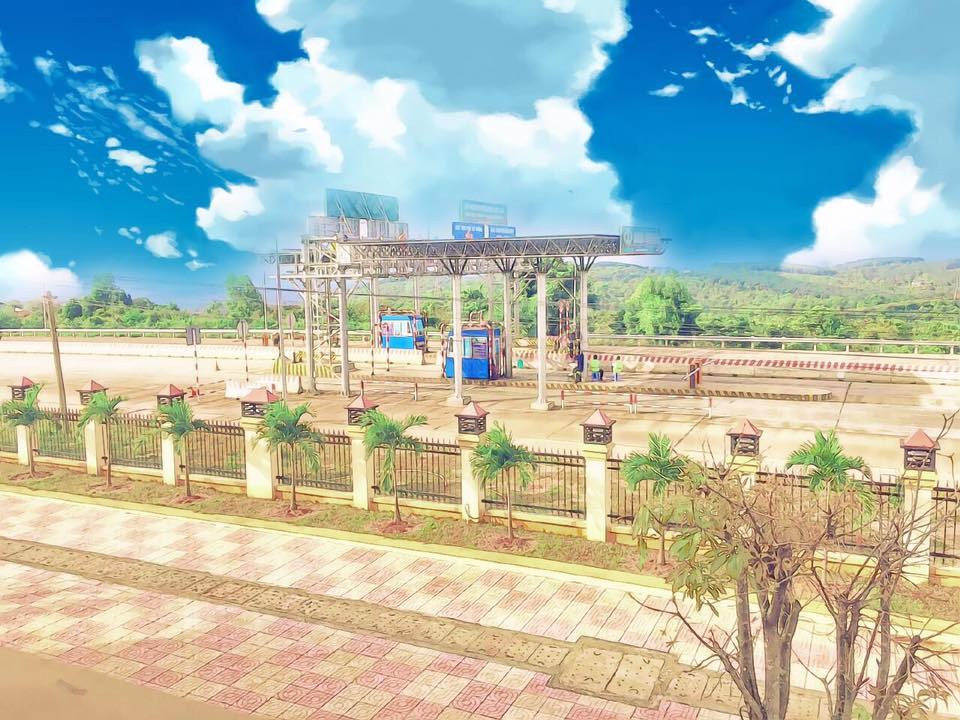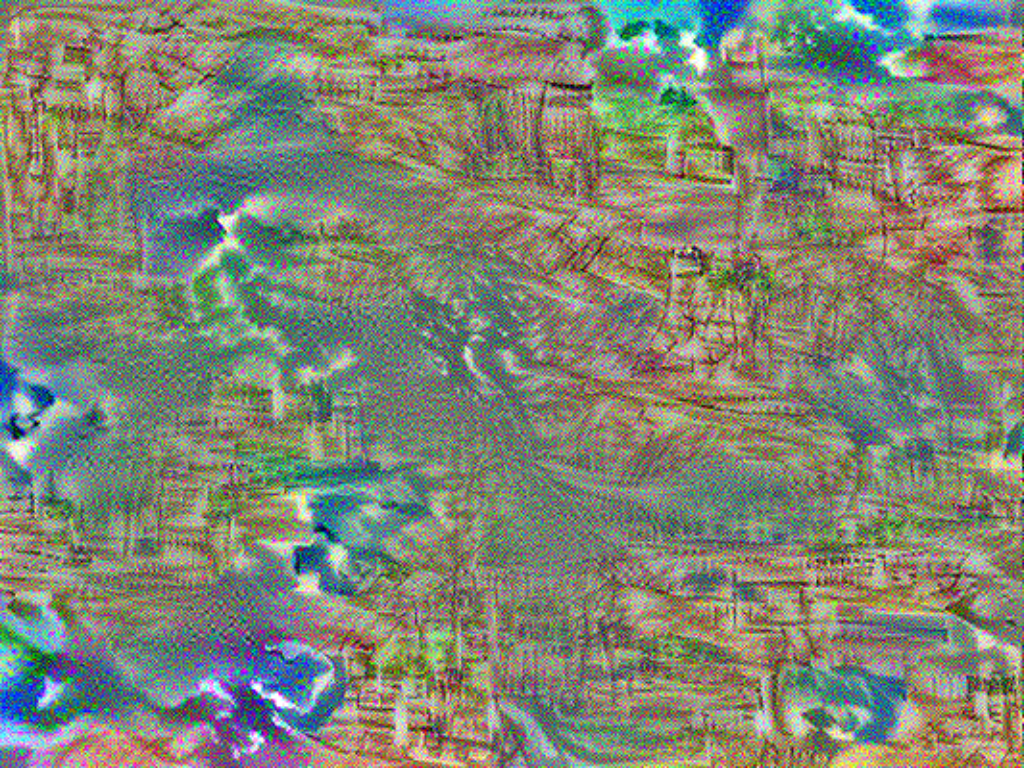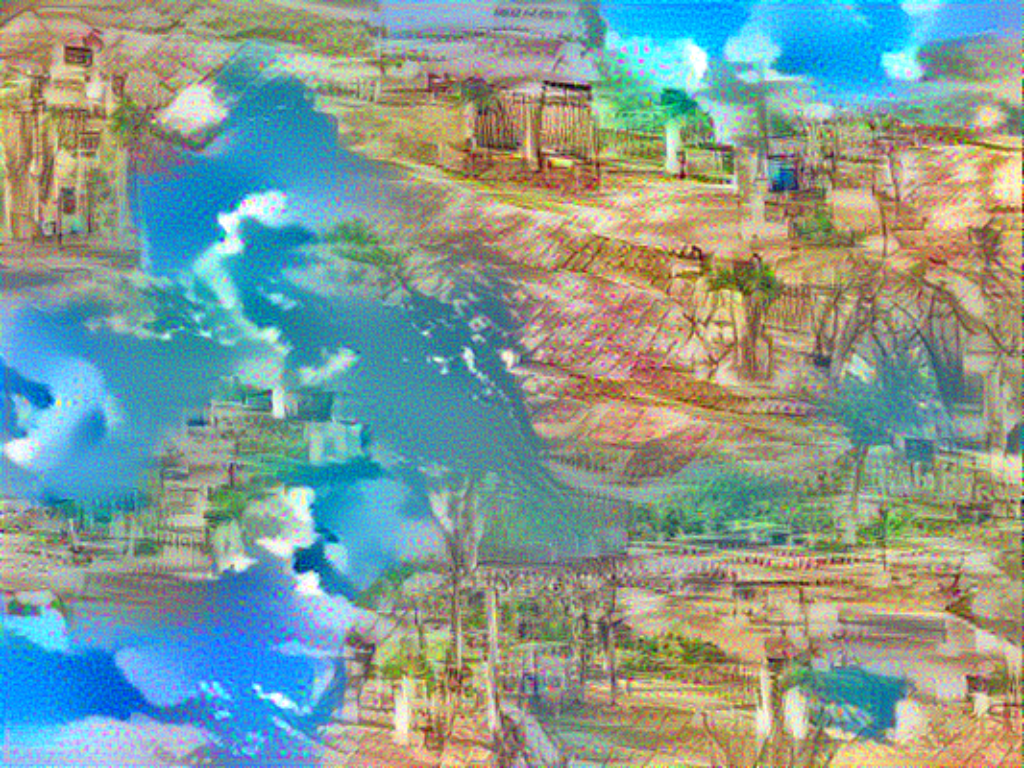Figure 7: Another example of output from the algorithm for the dataset of size one. Top-left: the reference transformed image; Top-right: the generated image after 15 epochs; Bottom-left: the generated image after 150 epochs; Bottom-right: the generated image after 1500 epochs.
Figure 8: The learning curve corresponding to 3 examples shown above.

As shown by Figures 5-7, the generated style images are able to capture some content information and style information of their corresponding reference EverFilter-styled images. Specifically, some portions of the generated style image come from their reference images with different degree of photometric and geometric invariance. Some portions of the generated images are distorted. One observation we would like to address is that the generated images and their corresponding reference images seem to have similar color composition, which seems to be an indication that our objective function is able to capture information about color composition of an image.

Because a style image should be a representative of all images in the same domain that have similar style, contents of images should have no effects in generating a style image. In the next subsection, we increase the size of dataset used in our proposed objective function so that the generated style image will be a more generalized version of an image that is Everfilter-styled.

2nd Attempt: Dataset of size 4, without masking

In this experiment, we want to see a style image generated from multiple reference files. We set the weighting factor that determines the contribution of each reference style image equally. Figure 9 below shows a generated style image, created based on 4 reference EverFilter-styled images. In this version of generated style image, it is still possible to see that some portions of the generated image come from the reference files. However, it is harder to indicate which reference file those portions correspond together.

Artistically, the style image generated based on multiple reference files seems to be more abstarct and harder to explain what is going on in the image. Here, we note that since the VGG-19 model requires a lot of memory for images' feature representations. As a result, we can use only maximum 4 images to generate style images.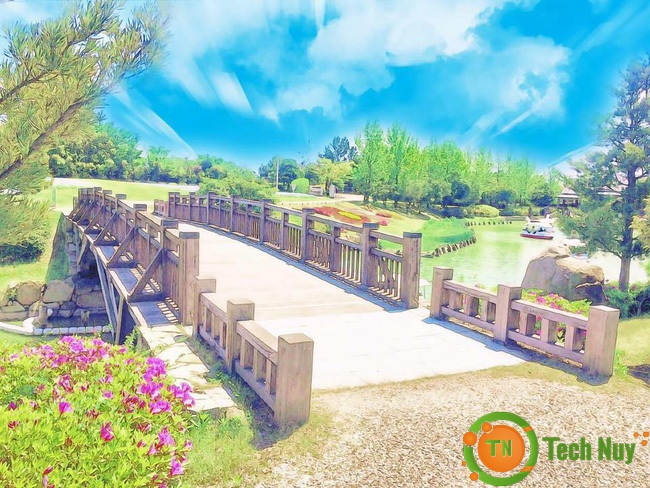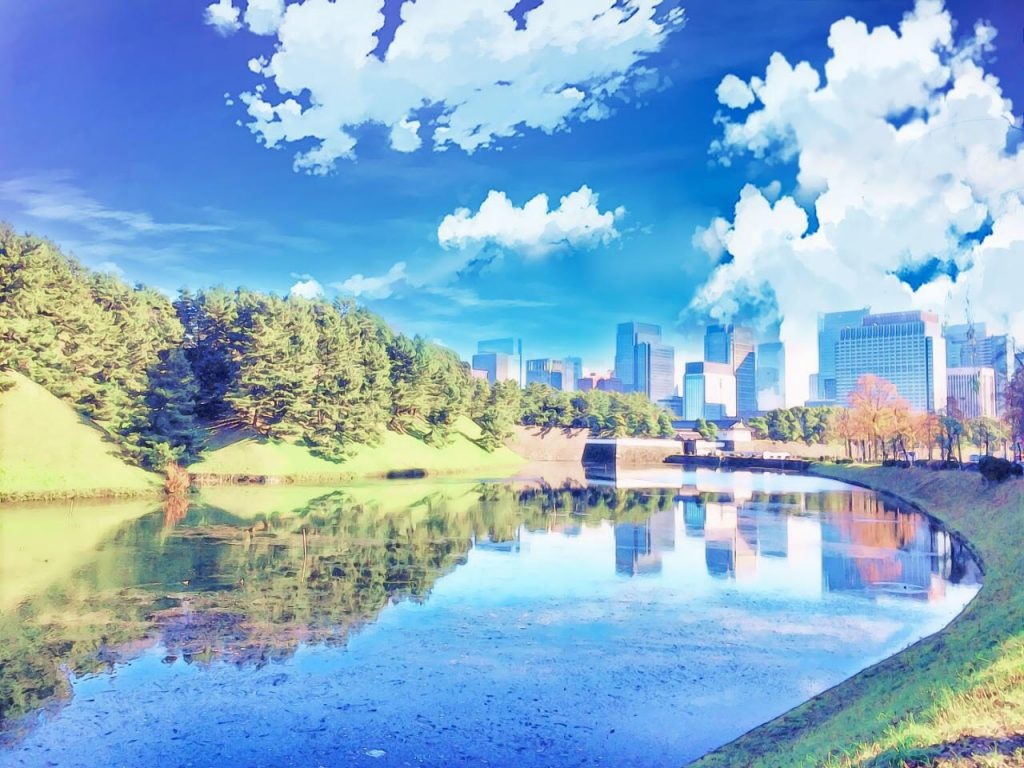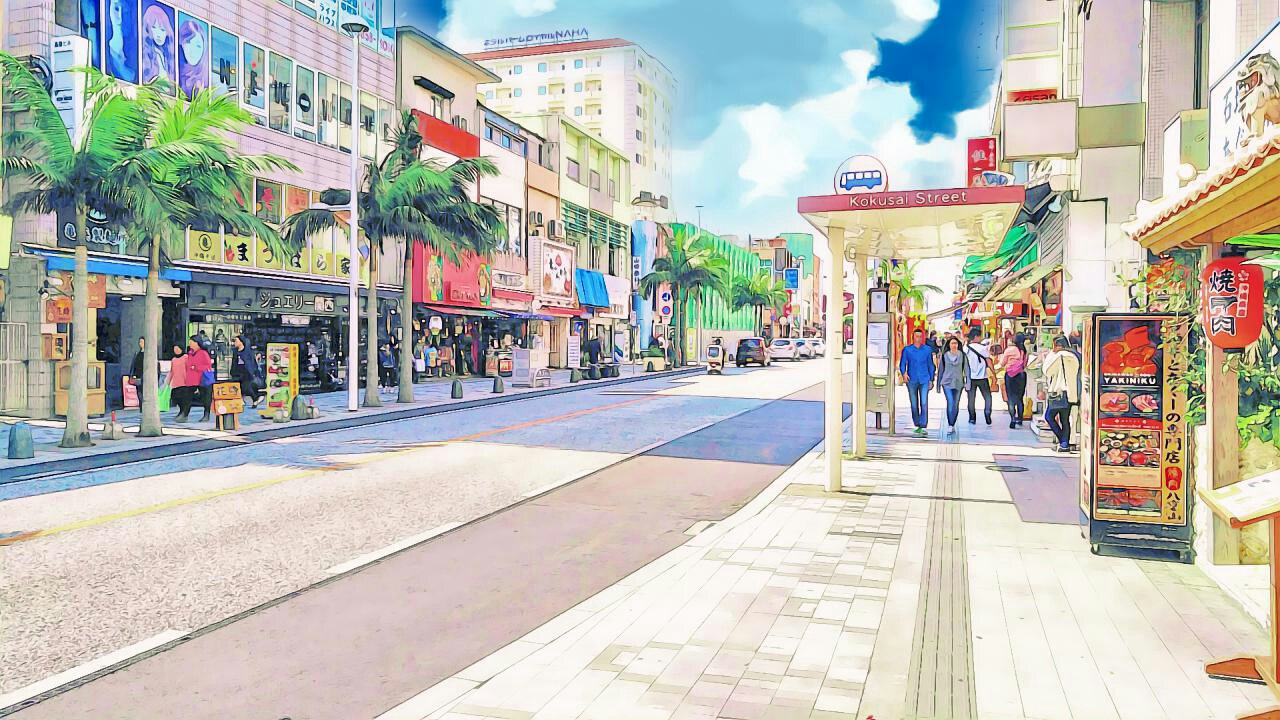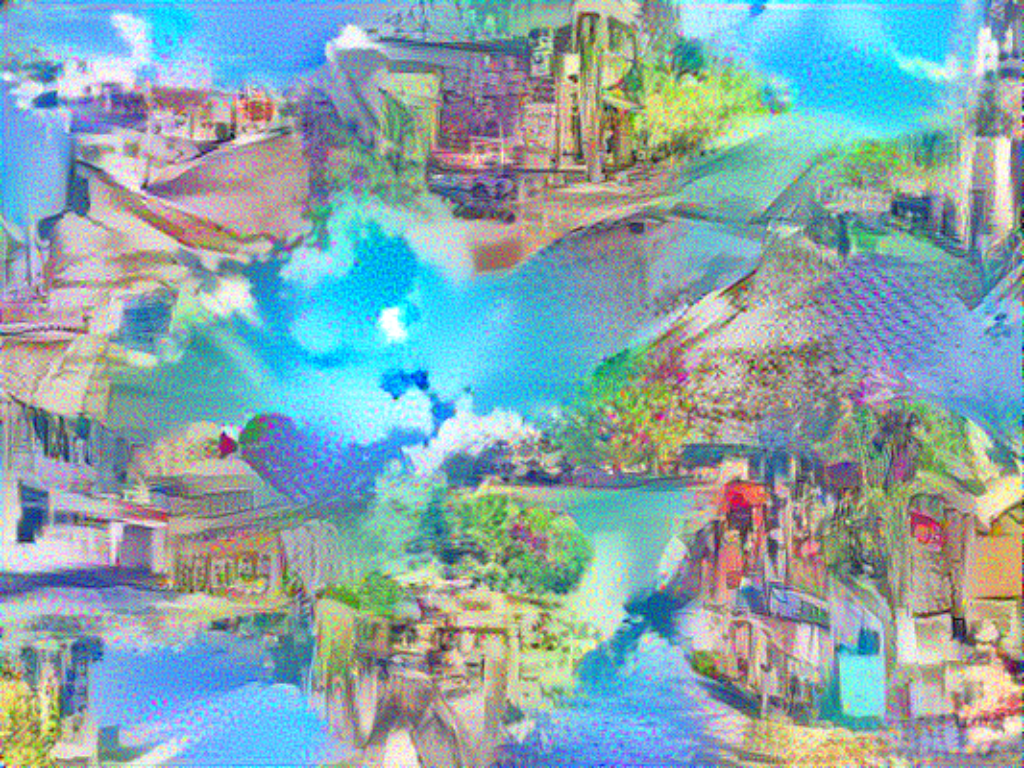Figure 9: An example of style image generated from 4 different reference files with equal contribution. Top 4 images: the reference EverFilter-styled images; Bottom: the generated style image from our algorithm.
Figure 10: The learning curve for the above example

3rd Attempt: Dataset of size 1, with masking

In this experiment, we are interested in generating a style based on different portion of the reference image separately because, arguably, one can claim that the background portion of an image has very different style from the foreground portion of an image. Here, we generated a style on foreground portion and background portion separately by applying a masking technique. For example, if we generate an image based on the background portion of the reference file, we calculate the Gram matrices as usual; however, we ignore values outside the mask region. Figure 11 shows an example of what our algorithm produced.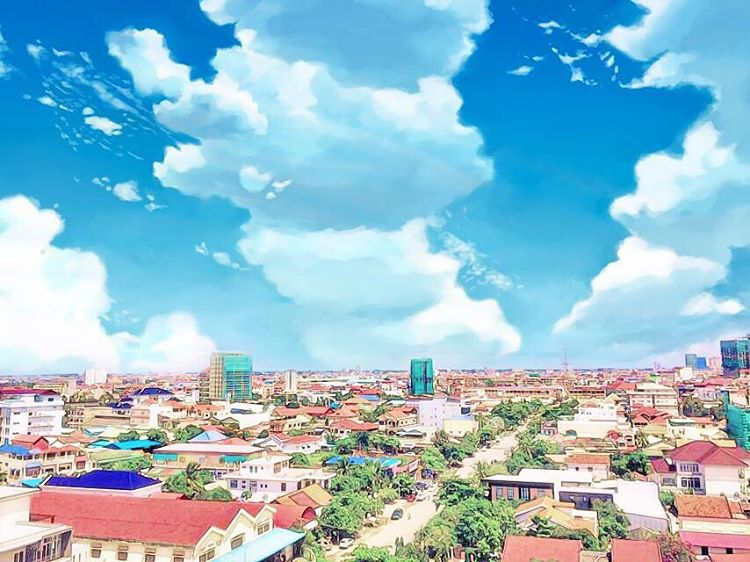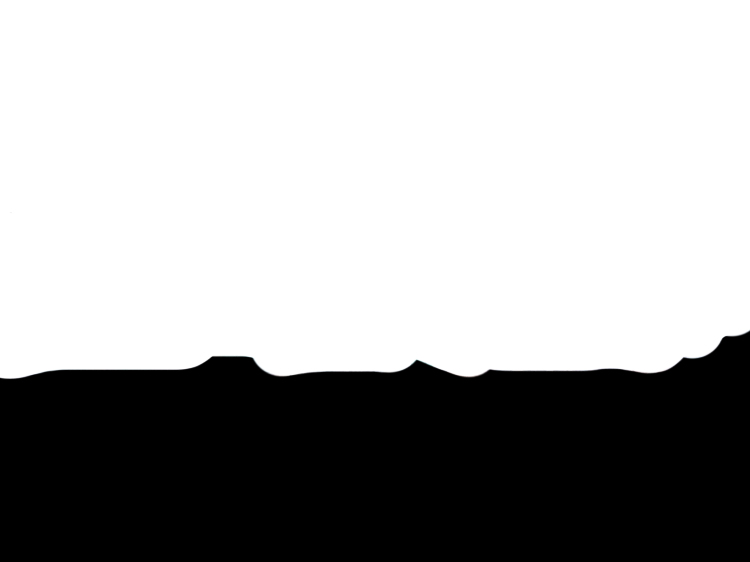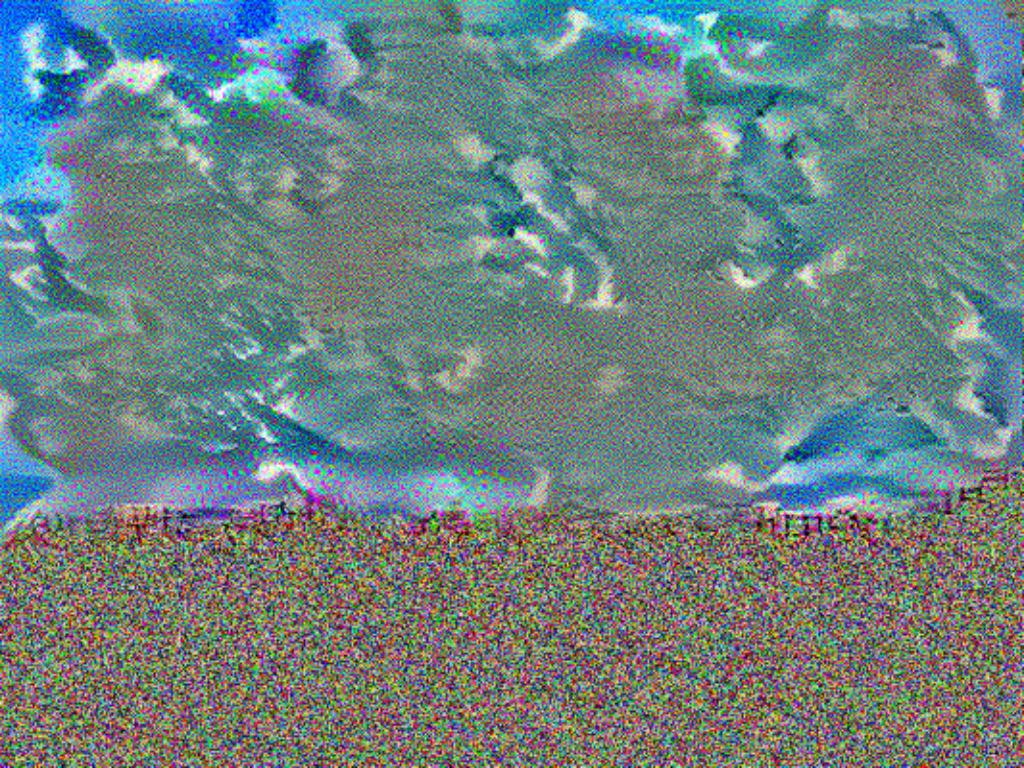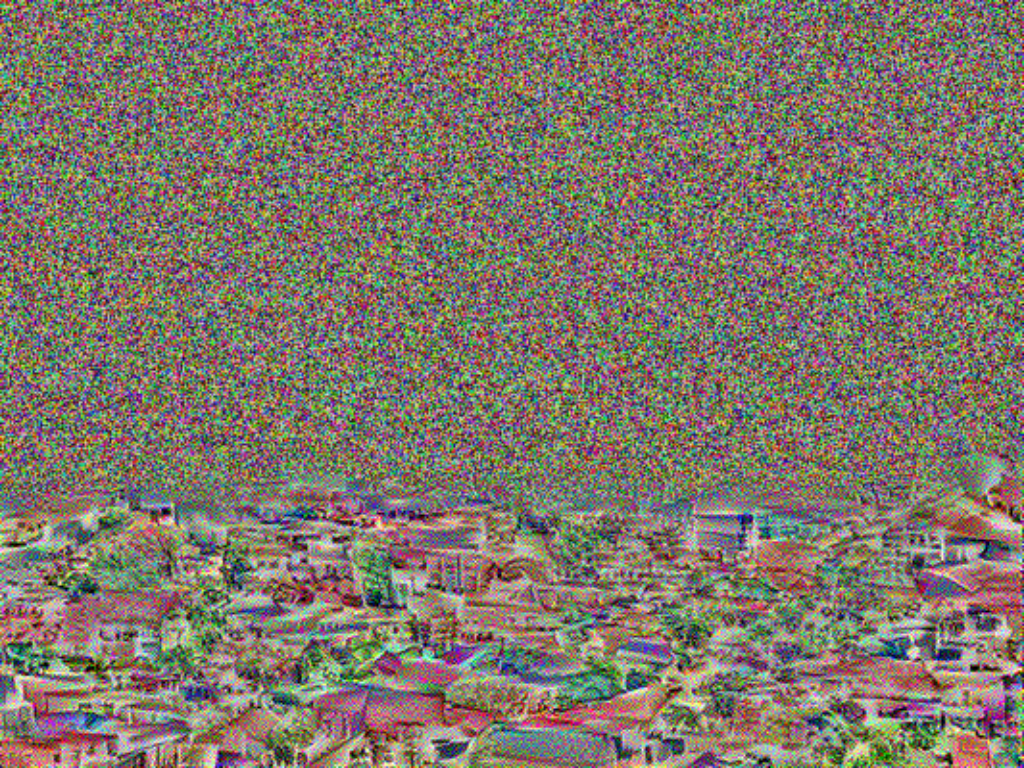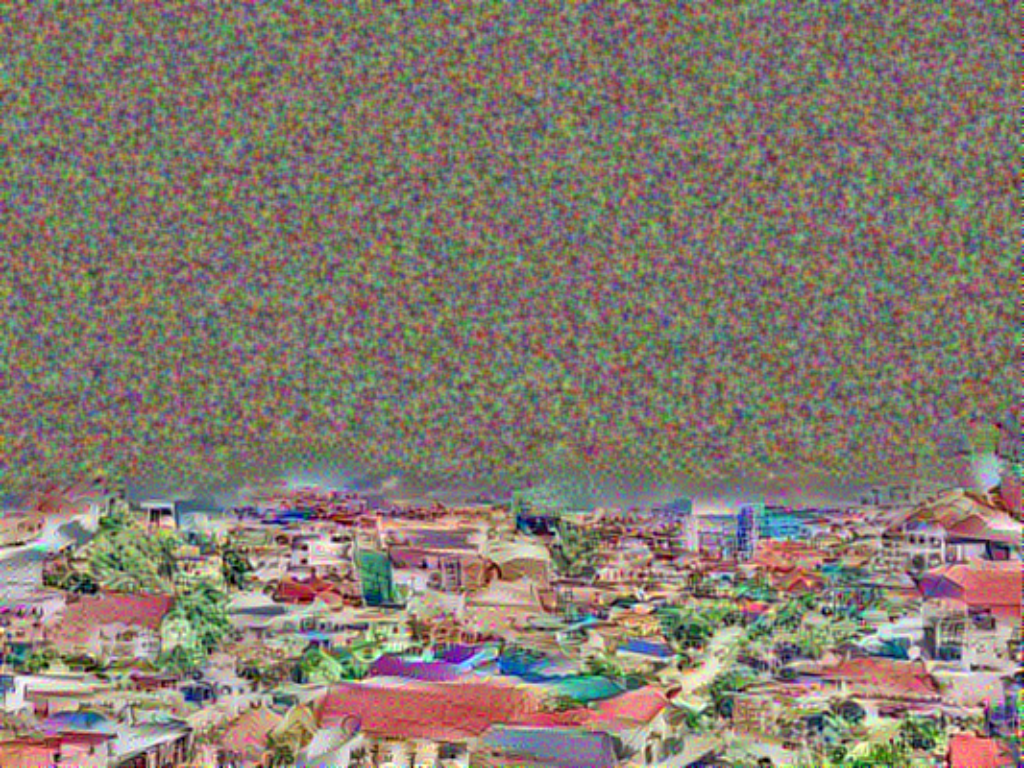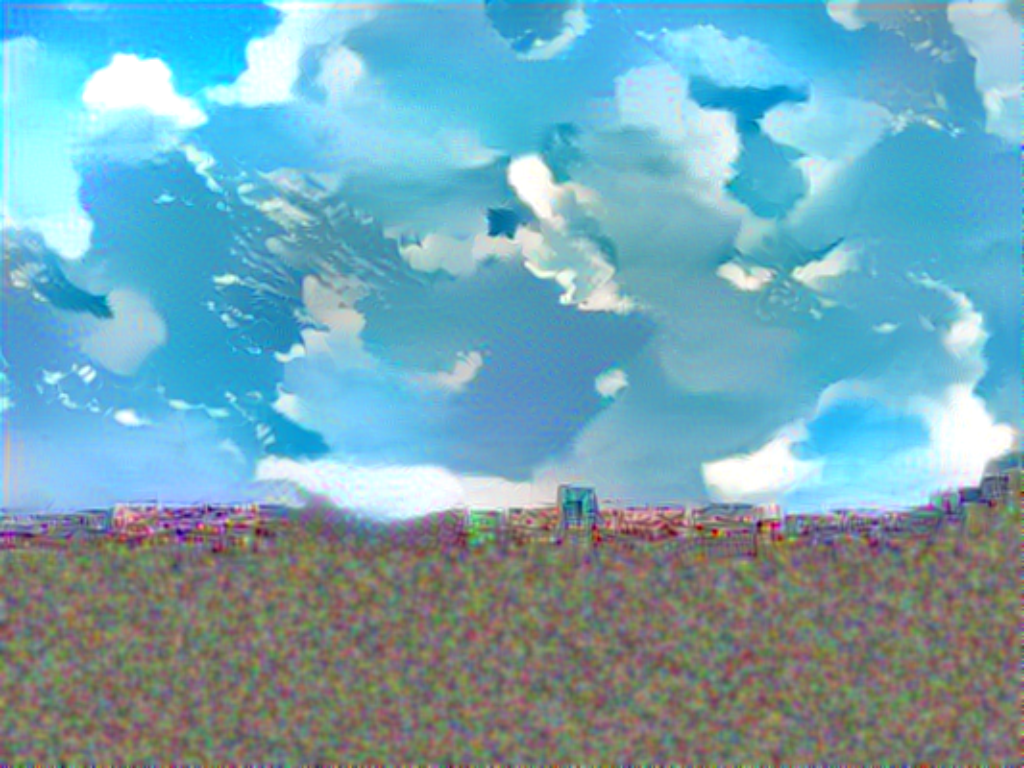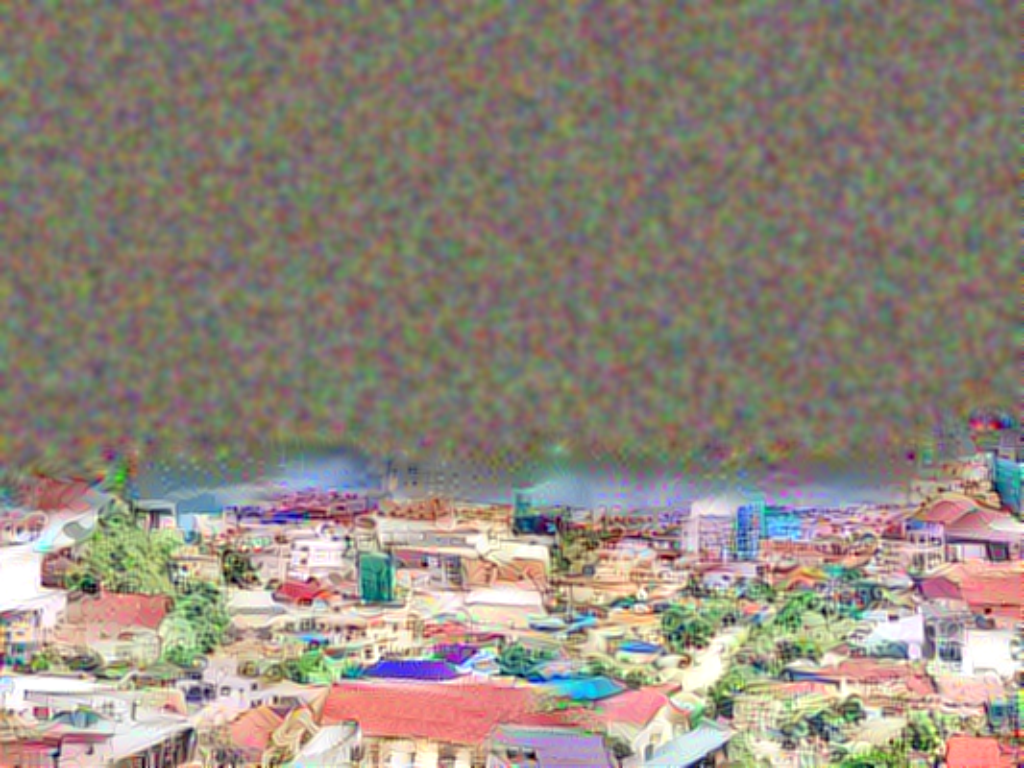Figure 11: An example of what our algorithm produced if the masking technique is applied. Top-left: the reference EverFilter-styled image; Top-right: the mask for background and foreground portions; Other left images: the generated images for background portion at 15, 150, and 1500 epochs; Other right images: the generated images for foreground portion at 15, 150, and 1500 epochs.
Figure 12: The learning curve of above example

Since the masking technique requires that the position of specific portion is the same for a generated image and a reference image, we cannot apply this masking tecnhique with multiple reference files, as the positions of portions with similar style must be the same. Even though this can be done with some kind of semantic image segmentation algorithm, it is out of the scope for this final project.

# Conclusion

From our experiments, we have better understanding of what contributes to a style of an image. Based on the definitions of style according to Image style transfer using convolutional neural networks that involves the Frobenius norm of the difference between two Gram matrices, style, as captured by Gram matrices, can be explained using a concept of probability distributions. For example, we can generate an image of sky with similar style to Everfilter-styled images by sampling from the distribution of Everfilter-styled sky images.

Using the concept of probability distributions, this finding has an important implication that we can generate any image with similar style if we have a way to construct such distribution. We believe that constructing a distribution of any image can be done with enough samples from that distribution.

As depicted by Figures 5, 6, 7, and 9, our finding has shown that if we construct distributions based on entire image, the generated images will be jumbled together. Instead, if we construct distributions based on only specific portions of an image, as illustrated in Figure 11, the generated image seems to more stylistically coherent and more visually interpretable. By using a masking technique, one can generate a style image that visualizes the characteristics of reference image files.

# Future Works

In future works, we would like to see the effects of different hyperparameters for the case of multiple reference image files. Also, we would like to see the experiments done on larger datasets. We are also interested in exploring different ways of defining style rather than using a Gram matrix. A recent paper Demystifying Neural Style Transfer explains that matching the Gram matrices of feature maps is equivalent to minimizing the Maximum Mean Discrepancy (MMD) with the second order polynomial kernel. We would like to relate our works to the concept of minimizing the Maximum Mean Discrepancy with different kernel function in the future research.

# Acknowledgements

This work used the Extreme Science and Engineering Discovery Environment (XSEDE), which is supported by National Science Foundation grant number OCI-1053575. Specifically, it used the Bridges system, which is supported by NSF award number ACI-1445606, at the Pittsburgh Supercomputing Center (PSC).

# References

1. Chan, Ethan, and Rishabh Bhargava. "Show, Divide and Neural: Weighted Style Transfer". N.p., 2016. Web. 2017.
2. Gatys, Leon A., Alexander S. Ecker, and Matthias Bethge. "A neural algorithm of artistic style." arXiv preprint arXiv:1508.06576 (2015).
3. Gatys, Leon A., Alexander S. Ecker, and Matthias Bethge. "Image style transfer using convolutional neural networks." Proceedings of the IEEE Conference on Computer Vision and Pattern Recognition. 2016.
4. J. Deng, A. Berg, S. Satheesh, H. Su, A. Khosla, and L. Fei-Fei. ILSVRC-2012, 2012. URL http://www.image-net.org/challenges/LSVRC/2012/.
5. Li, Yanghao, et al. "Demystifying Neural Style Transfer." arXiv preprint arXiv:1701.01036 (2017).
6. Luan, Fujun, et al. "Deep Photo Style Transfer." arXiv preprint arXiv:1703.07511 (2017).
7. Mahendran, Aravindh, and Andrea Vedaldi. "Understanding deep image representations by inverting them." Proceedings of the IEEE Conference on Computer Vision and Pattern Recognition. 2015.
8. Novak, Roman, and Yaroslav Nikulin. "Improving the neural algorithm of artistic style." arXiv preprint arXiv:1605.04603 (2016).
9. Nystrom, N. A., Levine, M. J., Roskies, R. Z., and Scott, J. R. 2015. Bridges: A Uniquely Flexible HPC Resource for New Communities and Data Analytics. In Proceedings of the 2015 Annual Conference on Extreme Science and Engineering Discovery Environment (St. Louis, MO, July 26-30, 2015). XSEDE15. ACM, New York, NY, USA. http://dx.doi.org/10.1145/2792745.2792775.
10. Simonyan, Karen, and Andrew Zisserman. "Very deep convolutional networks for large-scale image recognition." arXiv preprint arXiv:1409.1556 (2014).
11. Towns, J., Cockerill, T., Dahan, M., Foster, I., Gaither, K., Grimshaw, A., Hazlewood, V., Lathrop, S., Lifka, D., Peterson, G.D., Roskies, R., Scott, J.R. and Wilkens-Diehr, N. 2014. XSEDE: Accelerating Scientific Discovery. Computing in Science & Engineering. 16(5):62-74. http://doi.ieeecomputersociety.org/10.1109/MCSE.2014.80.
12. https://github.com/titu1994/Neural-Style-Transfer
13. https://github.com/fchollet/keras